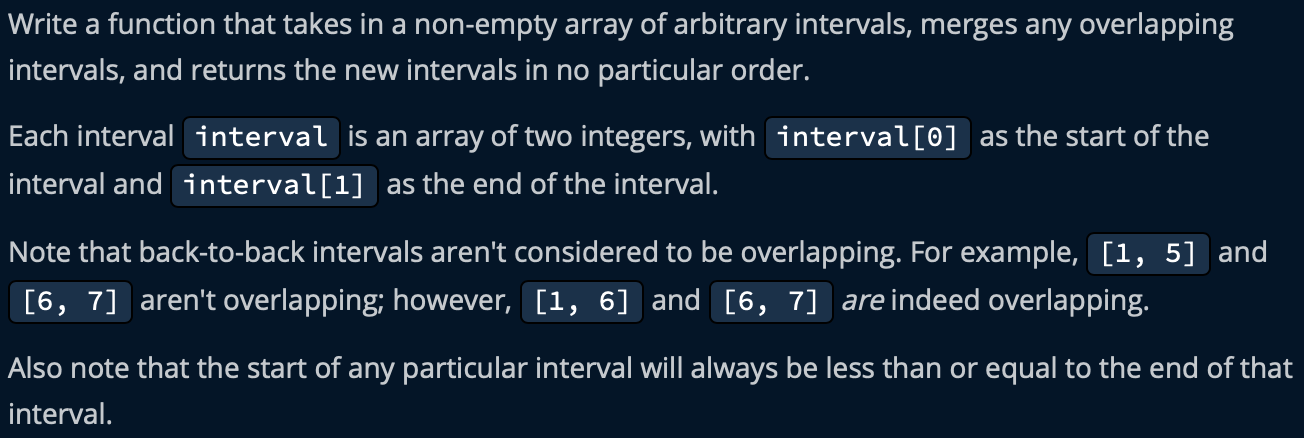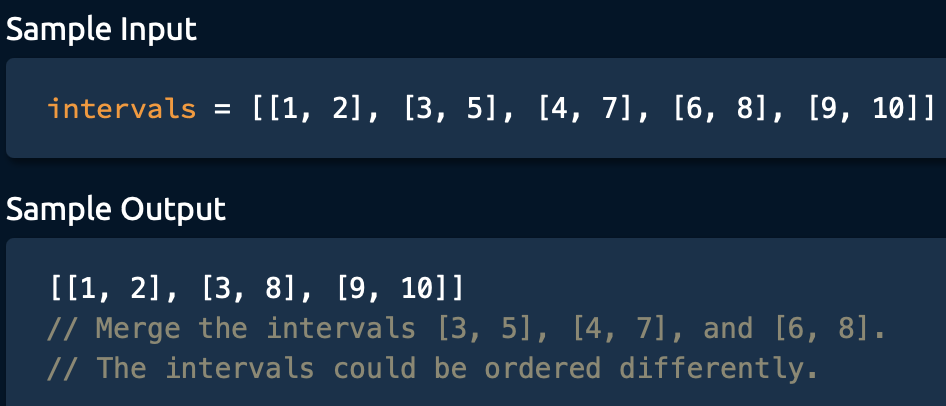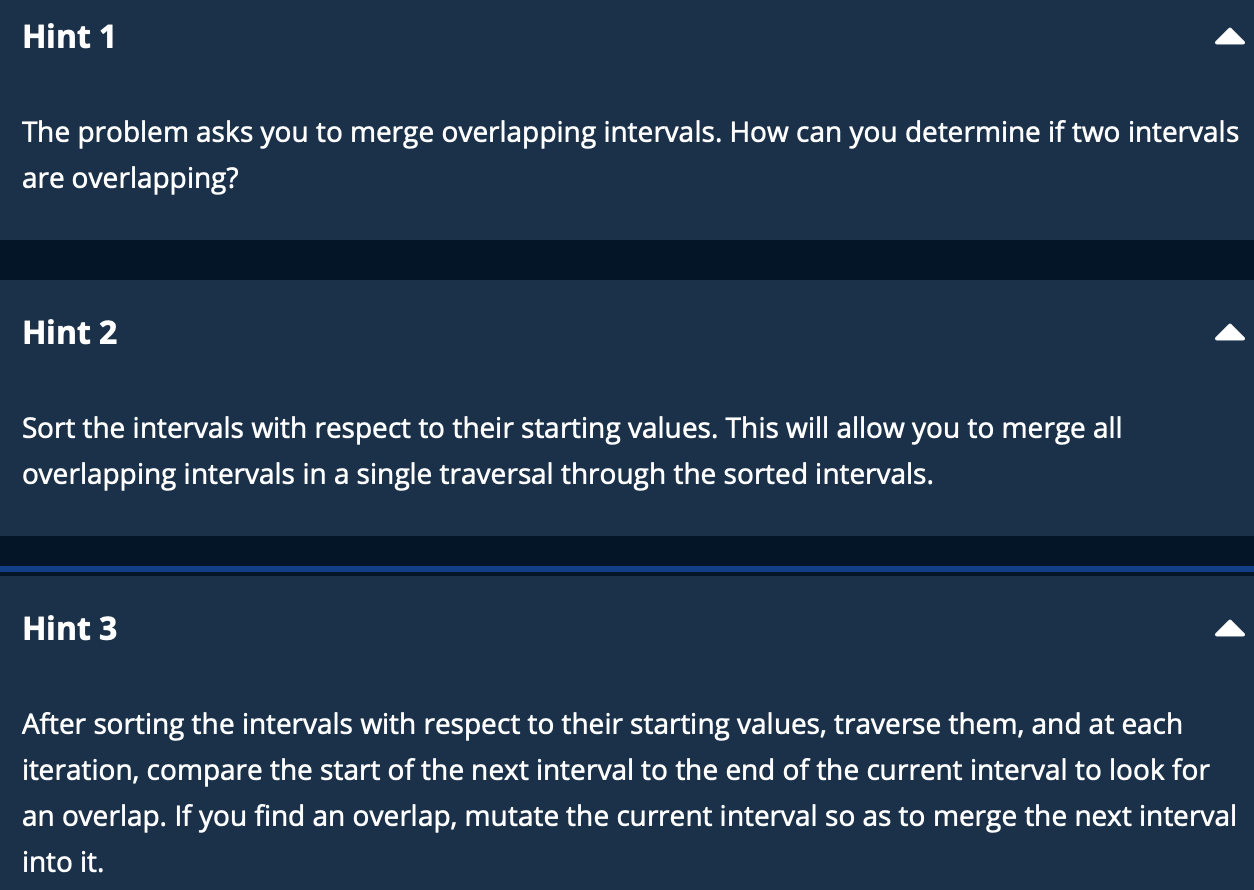5G时代下一个聚合的编程学习网

# Merge Overlapping Intervals

## Problem Statement## Sample example## Analysis

step 1: sort the intervals by their start value

step 2: check if the end value of the previous interval is larger than the start value of the latter interval## Code

```def mergeOverlappingIntervals(intervals):
# sort the intervals by startinf value.  O(nlogn)
sortedIntervals = sorted(intervals, key = lambda x: x)

mergedIntervals = []# space: O(n)
currentInterval = sortedIntervals#initialize the currentInterval as the first interval of the intervals
mergedIntervals.append(currentInterval)#initialize the mergedIntervals as the first interval of the intervals

for nextInterval in sortedIntervals:# for the first iteration, no update
_, currentIntervalEnd = currentInterval
nextIntervalStart, nextIntervalEnd = nextInterval

if currentIntervalEnd >= nextIntervalStart: # overlap found, update the end value of interval
currentInterval = max(currentIntervalEnd, nextIntervalEnd)
else: # no overlap, add the current(nextInterval) into the mergedIntervals array
currentInterval = nextInterval
mergedIntervals.append(currentInterval)
return mergedIntervals```

## Time and Space complexity

O(nlogn) time complexity for sorting

O(n) space complexity for store the mergedIntervals(upper bound, all intervals kept)